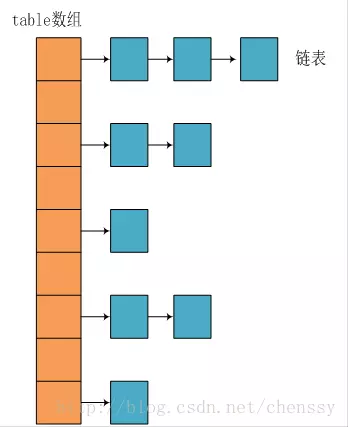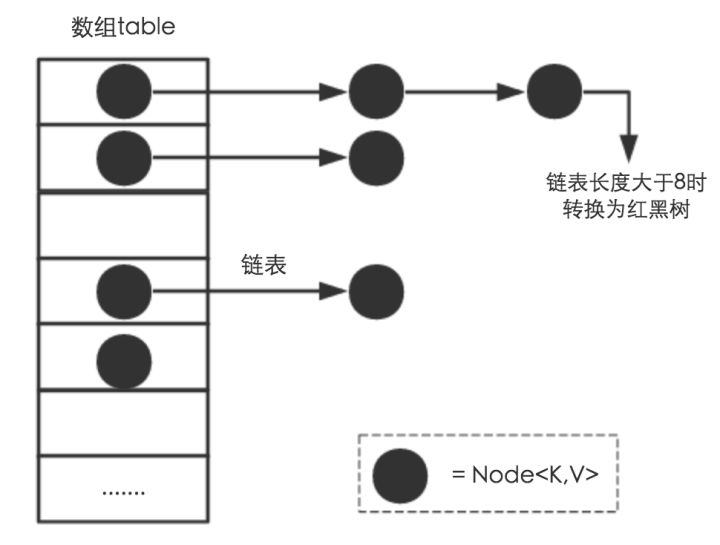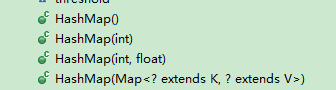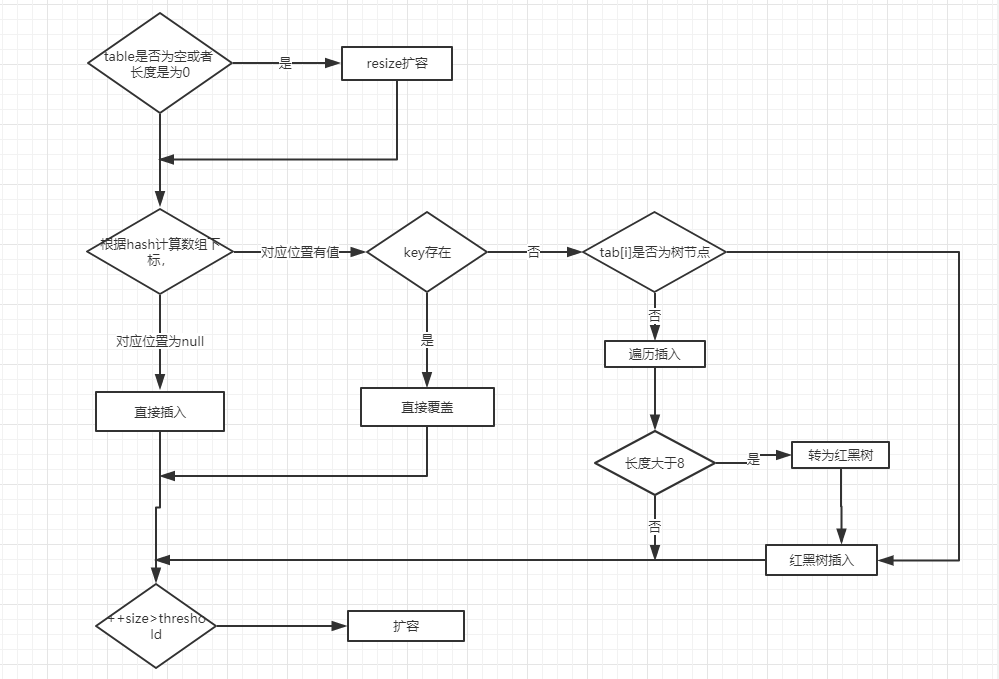HashMap 简介

HashMap 主要用来存放键值对，它基于哈希表的Map接口实现，是常用的Java集合之一。

JDK1.8 之前 HashMap 由 数组+链表 组成的，数组是 HashMap 的主体，链表则是主要为了解决哈希冲突而存在的（“拉链法”解决冲突）.JDK1.8 以后在解决哈希冲突时有了较大的变化，当链表长度大于阈值（默认为 8）时，将链表转化为红黑树，以减少搜索时间。

底层数据结构分析

JDK1.8之前

JDK1.8 之前 HashMap 底层是 数组和链表 结合在一起使用也就是 链表散列HashMap 通过 key 的 hashCode 经过扰动函数处理过后得到 hash 值，然后通过 `(n - 1) & hash` 判断当前元素存放的位置（这里的 n 指的是数组的长度），如果当前位置存在元素的话，就判断该元素与要存入的元素的 hash 值以及 key 是否相同，如果相同的话，直接覆盖，不相同就通过拉链法解决冲突。

JDK 1.8 HashMap 的 hash 方法源码:

JDK 1.8 的 hash方法 相比于 JDK 1.7 hash 方法更加简化，但是原理不变。

 1 2 3 4 5 6 7 `static` `final` `int` `hash(``Object` `key) {` `  ``int` `h;` `  ``// key.hashCode()：返回散列值也就是hashcode` `  ``// ^ ：按位异或` `  ``// >>>:无符号右移，忽略符号位，空位都以0补齐` `  ``return` `(key == ``null``) ? ``0` `: (h = key.hashCode()) ^ (h >>> ``16``);` `}`

 1 2 3 4 5 6 7 8 `static` `int hash(int h) {` `    ``// This function ensures that hashCodes that differ only by` `    ``// constant multiples at each bit position have a bounded` `    ``// number of collisions (approximately 8 at default load factor).`   `    ``h ^= (h >>> ``20``) ^ (h >>> ``12``);` `    ``return` `h ^ (h >>> ``7``) ^ (h >>> ``4``);` `}`JDK1.8之后1 2 3 4 5 6 7 8 9 10 11 12 13 14 15 16 17 18 19 20 21 22 23 24 25 26 27 28 `public` `class` `HashMap ``extends` `AbstractMap ``implements` `Map, Cloneable, Serializable {` `    ``// 序列号` `    ``private` `static` `final` `long` `serialVersionUID = 362498820763181265L;    ` `    ``// 默认的初始容量是16` `    ``static` `final` `int` `DEFAULT_INITIAL_CAPACITY = ``1` `<< ``4``;   ` `    ``// 最大容量` `    ``static` `final` `int` `MAXIMUM_CAPACITY = ``1` `<< ``30``; ` `    ``// 默认的填充因子` `    ``static` `final` `float` `DEFAULT_LOAD_FACTOR = ``0``.75f;` `    ``// 当桶(bucket)上的结点数大于这个值时会转成红黑树` `    ``static` `final` `int` `TREEIFY_THRESHOLD = ``8``; ` `    ``// 当桶(bucket)上的结点数小于这个值时树转链表` `    ``static` `final` `int` `UNTREEIFY_THRESHOLD = ``6``;` `    ``// 桶中结构转化为红黑树对应的table的最小大小` `    ``static` `final` `int` `MIN_TREEIFY_CAPACITY = ``64``;` `    ``// 存储元素的数组，总是2的幂次倍` `    ``transient` `Node[] table; ` `    ``// 存放具体元素的集` `    ``transient` `Set> entrySet;` `    ``// 存放元素的个数，注意这个不等于数组的长度。` `    ``transient` `int` `size;` `    ``// 每次扩容和更改map结构的计数器` `    ``transient` `int` `modCount;   ` `    ``// 临界值 当实际大小(容量*填充因子)超过临界值时，会进行扩容` `    ``int` `threshold;` `    ``// 填充因子` `    ``final` `float` `loadFactor;` `}`

• threshold

threshold = capacity * loadFactor，当Size>=threshold的时候，那么就要考虑对数组的扩增了，也就是说，这个的意思就是 衡量数组是否需要扩增的一个标准。

Node节点类源码：

 1 2 3 4 5 6 7 8 9 10 11 12 13 14 15 16 17 18 19 20 21 22 23 24 25 26 27 28 29 30 31 32 33 34 35 36 37 38 39 `// 继承自 Map.Entry` `static` `class` `Node ``implements` `Map.Entry {` `       ``final` `int` `hash;``// 哈希值，存放元素到hashmap中时用来与其他元素hash值比较` `       ``final` `K key;``//键` `       ``V value;``//值` `       ``// 指向下一个节点` `       ``Node next;` `       ``Node(``int` `hash, K key, V value, Node next) {` `            ``this``.hash = hash;` `            ``this``.key = key;` `            ``this``.value = value;` `            ``this``.next = next;` `        ``}` `        ``public` `final` `K getKey()        { ``return` `key; }` `        ``public` `final` `V getValue()      { ``return` `value; }` `        ``public` `final` `String toString() { ``return` `key + ``"="` `+ value; }` `        ``// 重写hashCode()方法` `        ``public` `final` `int` `hashCode() {` `            ``return` `Objects.hashCode(key) ^ Objects.hashCode(value);` `        ``}`   `        ``public` `final` `V setValue(V newValue) {` `            ``V oldValue = value;` `            ``value = newValue;` `            ``return` `oldValue;` `        ``}` `        ``// 重写 equals() 方法` `        ``public` `final` `boolean` `equals(Object o) {` `            ``if` `(o == ``this``)` `                ``return` `true``;` `            ``if` `(o ``instanceof` `Map.Entry) {` `                ``Map.Entry e = (Map.Entry)o;` `                ``if` `(Objects.equals(key, e.getKey()) &&` `                    ``Objects.equals(value, e.getValue()))` `                    ``return` `true``;` `            ``}` `            ``return` `false``;` `        ``}` `}`

 1 2 3 4 5 6 7 8 9 10 11 12 13 14 15 16 `static` `final` `class` `TreeNode ``extends` `LinkedHashMap.Entry {` `        ``TreeNode parent;  ``// 父` `        ``TreeNode left;    ``// 左` `        ``TreeNode right;   ``// 右` `        ``TreeNode prev;    ``// needed to unlink next upon deletion` `        ``boolean` `red;           ``// 判断颜色` `        ``TreeNode(``int` `hash, K key, V val, Node next) {` `            ``super``(hash, key, val, next);` `        ``}` `        ``// 返回根节点` `        ``final` `TreeNode root() {` `            ``for` `(TreeNode r = ``this``, p;;) {` `                ``if` `((p = r.parent) == ``null``)` `                    ``return` `r;` `                ``r = p;` `       ``}`

HashMap源码分析

构造方法1 2 3 4 5 6 7 8 9 10 11 12 13 14 15 16 17 18 19 20 21 22 23 24 25 26 27 `// 默认构造函数。` `public` `More ...HashMap() {` `    ``this``.loadFactor = DEFAULT_LOAD_FACTOR; ``// all   other fields defaulted` ` ``}`   ` ``// 包含另一个“Map”的构造函数` ` ``public` `More ...HashMap(Map m) {` `     ``this``.loadFactor = DEFAULT_LOAD_FACTOR;` `     ``putMapEntries(m, ``false``);``//下面会分析到这个方法` ` ``}`   ` ``// 指定“容量大小”的构造函数` ` ``public` `More ...HashMap(``int` `initialCapacity) {` `     ``this``(initialCapacity, DEFAULT_LOAD_FACTOR);` ` ``}`   ` ``// 指定“容量大小”和“加载因子”的构造函数` ` ``public` `More ...HashMap(``int` `initialCapacity, ``float` `loadFactor) {` `     ``if` `(initialCapacity < ``0``)` `         ``throw` `new` `IllegalArgumentException(``"Illegal initial capacity: "` `+ initialCapacity);` `     ``if` `(initialCapacity > MAXIMUM_CAPACITY)` `         ``initialCapacity = MAXIMUM_CAPACITY;` `     ``if` `(loadFactor <= ``0` `|| Float.isNaN(loadFactor))` `         ``throw` `new` `IllegalArgumentException(``"Illegal load factor: "` `+ loadFactor);` `     ``this``.loadFactor = loadFactor;` `     ``this``.threshold = tableSizeFor(initialCapacity);` ` ``}`

putMapEntries方法：

 1 2 3 4 5 6 7 8 9 10 11 12 13 14 15 16 17 18 19 20 21 22 23 24 `final` `void` `putMapEntries(Map m, ``boolean` `evict) {` `    ``int` `s = m.size();` `    ``if` `(s > ``0``) {` `        ``// 判断table是否已经初始化` `        ``if` `(table == ``null``) { ``// pre-size` `            ``// 未初始化，s为m的实际元素个数` `            ``float` `ft = ((``float``)s / loadFactor) + ``1``.0F;` `            ``int` `t = ((ft < (``float``)MAXIMUM_CAPACITY) ?` `                    ``(``int``)ft : MAXIMUM_CAPACITY);` `            ``// 计算得到的t大于阈值，则初始化阈值` `            ``if` `(t > threshold)` `                ``threshold = tableSizeFor(t);` `        ``}` `        ``// 已初始化，并且m元素个数大于阈值，进行扩容处理` `        ``else` `if` `(s > threshold)` `            ``resize();` `        ``// 将m中的所有元素添加至HashMap中` `        ``for` `(Map.Entry e : m.entrySet()) {` `            ``K key = e.getKey();` `            ``V value = e.getValue();` `            ``putVal(hash(key), key, value, ``false``, evict);` `        ``}` `    ``}` `}`

put方法

HashMap只提供了put用于添加元素，putVal方法只是给put方法调用的一个方法，并没有提供给用户使用。

• ①如果定位到的数组位置没有元素 就直接插入。
• ②如果定位到的数组位置有元素就和要插入的 key 比较，如果key相同就直接覆盖，如果 key 不相同，就判断 p 是否是一个树节点，如果是就调用 `e = ((TreeNode<K,V>)p).putTreeVal(this, tab, hash, key, value) `将元素添加进入。如果不是就遍历链表插入。1 2 3 4 5 6 7 8 9 10 11 12 13 14 15 16 17 18 19 20 21 22 23 24 25 26 27 28 29 30 31 32 33 34 35 36 37 38 39 40 41 42 43 44 45 46 47 48 49 50 51 52 53 54 55 56 57 58 59 60 61 62 63 64 65 66 67 68 69 70 71 `public` `V put(K key, V value) {` `    ``return` `putVal(hash(key), key, value, ``false``, ``true``);` `}`   `final` `V putVal(``int` `hash, K key, V value, ``boolean` `onlyIfAbsent,` `                   ``boolean` `evict) {` `    ``Node[] tab; Node p; ``int` `n, i;` `    ``// table未初始化或者长度为0，进行扩容` `    ``if` `((tab = table) == ``null` `|| (n = tab.length) == ``0``)` `        ``n = (tab = resize()).length;` `    ``// (n - 1) & hash 确定元素存放在哪个桶中，桶为空，新生成结点放入桶中(此时，这个结点是放在数组中)` `    ``if` `((p = tab[i = (n - ``1``) & hash]) == ``null``)` `        ``tab[i] = newNode(hash, key, value, ``null``);` `    ``// 桶中已经存在元素` `    ``else` `{` `        ``Node e; K k;` `        ``// 比较桶中第一个元素(数组中的结点)的hash值相等，key相等` `        ``if` `(p.hash == hash &&` `            ``((k = p.key) == key || (key != ``null` `&& key.equals(k))))` `                ``// 将第一个元素赋值给e，用e来记录` `                ``e = p;` `        ``// hash值不相等，即key不相等；为红黑树结点` `        ``else` `if` `(p ``instanceof` `TreeNode)` `            ``// 放入树中` `            ``e = ((TreeNode)p).putTreeVal(``this``, tab, hash, key, value);` `        ``// 为链表结点` `        ``else` `{` `            ``// 在链表最末插入结点` `            ``for` `(``int` `binCount = ``0``; ; ++binCount) {` `                ``// 到达链表的尾部` `                ``if` `((e = p.next) == ``null``) {` `                    ``// 在尾部插入新结点` `                    ``p.next = newNode(hash, key, value, ``null``);` `                    ``// 结点数量达到阈值，转化为红黑树` `                    ``if` `(binCount >= TREEIFY_THRESHOLD - ``1``) ``// -1 for 1st` `                        ``treeifyBin(tab, hash);` `                    ``// 跳出循环` `                    ``break``;` `                ``}` `                ``// 判断链表中结点的key值与插入的元素的key值是否相等` `                ``if` `(e.hash == hash &&` `                    ``((k = e.key) == key || (key != ``null` `&& key.equals(k))))` `                    ``// 相等，跳出循环` `                    ``break``;` `                ``// 用于遍历桶中的链表，与前面的e = p.next组合，可以遍历链表` `                ``p = e;` `            ``}` `        ``}` `        ``// 表示在桶中找到key值、hash值与插入元素相等的结点` `        ``if` `(e != ``null``) { ` `            ``// 记录e的value` `            ``V oldValue = e.value;` `            ``// onlyIfAbsent为false或者旧值为null` `            ``if` `(!onlyIfAbsent || oldValue == ``null``)` `                ``//用新值替换旧值` `                ``e.value = value;` `            ``// 访问后回调` `            ``afterNodeAccess(e);` `            ``// 返回旧值` `            ``return` `oldValue;` `        ``}` `    ``}` `    ``// 结构性修改` `    ``++modCount;` `    ``// 实际大小大于阈值则扩容` `    ``if` `(++size > threshold)` `        ``resize();` `    ``// 插入后回调` `    ``afterNodeInsertion(evict);` `    ``return` `null``;` `}`

• ①如果定位到的数组位置没有元素 就直接插入。
• ②如果定位到的数组位置有元素，遍历以这个元素为头结点的链表，依次和插入的key比较，如果key相同就直接覆盖，不同就采用头插法插入元素。
 1 2 3 4 5 6 7 8 9 10 11 12 13 14 15 16 17 18 19 20 21 22 `public` `V put(K key, V value)` `    ``if` `(table == EMPTY_TABLE) { ` `    ``inflateTable(threshold); ` `}  ` `    ``if` `(key == ``null``)` `        ``return` `putForNullKey(value);` `    ``int` `hash = hash(key);` `    ``int` `i = indexFor(hash, table.length);` `    ``for` `(Entry e = table[i]; e != ``null``; e = e.next) { ``// 先遍历` `        ``Object k;` `        ``if` `(e.hash == hash && ((k = e.key) == key || key.equals(k))) {` `            ``V oldValue = e.value;` `            ``e.value = value;` `            ``e.recordAccess(``this``);` `            ``return` `oldValue; ` `        ``}` `    ``}`   `    ``modCount++;` `    ``addEntry(hash, key, value, i);  ``// 再插入` `    ``return` `null``;` `}`

get方法

 1 2 3 4 5 6 7 8 9 10 11 12 13 14 15 16 17 18 19 20 21 22 23 24 25 26 27 28 `public` `V get(Object key) {` `    ``Node e;` `    ``return` `(e = getNode(hash(key), key)) == ``null` `? ``null` `: e.value;` `}`   `final` `Node getNode(``int` `hash, Object key) {` `    ``Node[] tab; Node first, e; ``int` `n; K k;` `    ``if` `((tab = table) != ``null` `&& (n = tab.length) > ``0` `&&` `        ``(first = tab[(n - ``1``) & hash]) != ``null``) {` `        ``// 数组元素相等` `        ``if` `(first.hash == hash && ``// always check first node` `            ``((k = first.key) == key || (key != ``null` `&& key.equals(k))))` `            ``return` `first;` `        ``// 桶中不止一个节点` `        ``if` `((e = first.next) != ``null``) {` `            ``// 在树中get` `            ``if` `(first ``instanceof` `TreeNode)` `                ``return` `((TreeNode)first).getTreeNode(hash, key);` `            ``// 在链表中get` `            ``do` `{` `                ``if` `(e.hash == hash &&` `                    ``((k = e.key) == key || (key != ``null` `&& key.equals(k))))` `                    ``return` `e;` `            ``} ``while` `((e = e.next) != ``null``);` `        ``}` `    ``}` `    ``return` `null``;` `}`

resize方法

 1 2 3 4 5 6 7 8 9 10 11 12 13 14 15 16 17 18 19 20 21 22 23 24 25 26 27 28 29 30 31 32 33 34 35 36 37 38 39 40 41 42 43 44 45 46 47 48 49 50 51 52 53 54 55 56 57 58 59 60 61 62 63 64 65 66 67 68 69 70 71 72 73 74 75 76 77 78 79 80 `final` `Node[] resize() {` `    ``Node[] oldTab = table;` `    ``int` `oldCap = (oldTab == ``null``) ? ``0` `: oldTab.length;` `    ``int` `oldThr = threshold;` `    ``int` `newCap, newThr = ``0``;` `    ``if` `(oldCap > ``0``) {` `        ``// 超过最大值就不再扩充了，就只好随你碰撞去吧` `        ``if` `(oldCap >= MAXIMUM_CAPACITY) {` `            ``threshold = Integer.MAX_VALUE;` `            ``return` `oldTab;` `        ``}` `        ``// 没超过最大值，就扩充为原来的2倍` `        ``else` `if` `((newCap = oldCap << ``1``) < MAXIMUM_CAPACITY && oldCap >= DEFAULT_INITIAL_CAPACITY)` `            ``newThr = oldThr << ``1``; ``// double threshold` `    ``}` `    ``else` `if` `(oldThr > ``0``) ``// initial capacity was placed in threshold` `        ``newCap = oldThr;` `    ``else` `{ ` `        ``signifies using defaults` `        ``newCap = DEFAULT_INITIAL_CAPACITY;` `        ``newThr = (``int``)(DEFAULT_LOAD_FACTOR * DEFAULT_INITIAL_CAPACITY);` `    ``}` `    ``// 计算新的resize上限` `    ``if` `(newThr == ``0``) {` `        ``float` `ft = (``float``)newCap * loadFactor;` `        ``newThr = (newCap < MAXIMUM_CAPACITY && ft < (``float``)MAXIMUM_CAPACITY ? (``int``)ft : Integer.MAX_VALUE);` `    ``}` `    ``threshold = newThr;` `    ``@SuppressWarnings``({``"rawtypes"``,``"unchecked"``})` `        ``Node[] newTab = (Node[])``new` `Node[newCap];` `    ``table = newTab;` `    ``if` `(oldTab != ``null``) {` `        ``// 把每个bucket都移动到新的buckets中` `        ``for` `(``int` `j = ``0``; j < oldCap; ++j) {` `            ``Node e;` `            ``if` `((e = oldTab[j]) != ``null``) {` `                ``oldTab[j] = ``null``;` `                ``if` `(e.next == ``null``)` `                    ``newTab[e.hash & (newCap - ``1``)] = e;` `                ``else` `if` `(e ``instanceof` `TreeNode)` `                    ``((TreeNode)e).split(``this``, newTab, j, oldCap);` `                ``else` `{ ` `                    ``Node loHead = ``null``, loTail = ``null``;` `                    ``Node hiHead = ``null``, hiTail = ``null``;` `                    ``Node next;` `                    ``do` `{` `                        ``next = e.next;` `                        ``// 原索引` `                        ``if` `((e.hash & oldCap) == ``0``) {` `                            ``if` `(loTail == ``null``)` `                                ``loHead = e;` `                            ``else` `                                ``loTail.next = e;` `                            ``loTail = e;` `                        ``}` `                        ``// 原索引+oldCap` `                        ``else` `{` `                            ``if` `(hiTail == ``null``)` `                                ``hiHead = e;` `                            ``else` `                                ``hiTail.next = e;` `                            ``hiTail = e;` `                        ``}` `                    ``} ``while` `((e = next) != ``null``);` `                    ``// 原索引放到bucket里` `                    ``if` `(loTail != ``null``) {` `                        ``loTail.next = ``null``;` `                        ``newTab[j] = loHead;` `                    ``}` `                    ``// 原索引+oldCap放到bucket里` `                    ``if` `(hiTail != ``null``) {` `                        ``hiTail.next = ``null``;` `                        ``newTab[j + oldCap] = hiHead;` `                    ``}` `                ``}` `            ``}` `        ``}` `    ``}` `    ``return` `newTab;` `}`

HashMap常用方法测试

 1 2 3 4 5 6 7 8 9 10 11 12 13 14 15 16 17 18 19 20 21 22 23 24 25 26 27 28 29 30 31 32 33 34 35 36 37 38 39 40 41 42 43 44 45 46 47 48 49 50 51 52 53 54 55 56 57 58 59 60 61 62 63 64 65 66 67 68 69 70 `package` `map;`   `import` `java.util.Collection;` `import` `java.util.HashMap;` `import` `java.util.Set;`   `public` `class` `HashMapDemo {`   `    ``public` `static` `void` `main(String[] args) {` `        ``HashMap map = ``new` `HashMap();` `        ``// 键不能重复，值可以重复` `        ``map.put(``"san"``, ``"张三"``);` `        ``map.put(``"si"``, ``"李四"``);` `        ``map.put(``"wu"``, ``"王五"``);` `        ``map.put(``"wang"``, ``"老王"``);` `        ``map.put(``"wang"``, ``"老王2"``);``// 老王被覆盖` `        ``map.put(``"lao"``, ``"老王"``);` `        ``System.out.println(``"-------直接输出hashmap:-------"``);` `        ``System.out.println(map);` `        ``/**` `         ``* 遍历HashMap` `         ``*/` `        ``// 1.获取Map中的所有键` `        ``System.out.println(``"-------foreach获取Map中所有的键:------"``);` `        ``Set keys = map.keySet();` `        ``for` `(String key : keys) {` `            ``System.out.print(key+``"  "``);` `        ``}` `        ``System.out.println();``//换行` `        ``// 2.获取Map中所有值` `        ``System.out.println(``"-------foreach获取Map中所有的值:------"``);` `        ``Collection values = map.values();` `        ``for` `(String value : values) {` `            ``System.out.print(value+``"  "``);` `        ``}` `        ``System.out.println();``//换行` `        ``// 3.得到key的值的同时得到key所对应的值` `        ``System.out.println(``"-------得到key的值的同时得到key所对应的值:-------"``);` `        ``Set keys2 = map.keySet();` `        ``for` `(String key : keys2) {` `            ``System.out.print(key + ``"："` `+ map.get(key)+``"   "``);`   `        ``}` `        ``/**` `         ``* 另外一种不常用的遍历方式` `         ``*/` `        ``// 当我调用put(key,value)方法的时候，首先会把key和value封装到` `        ``// Entry这个静态内部类对象中，把Entry对象再添加到数组中，所以我们想获取` `        ``// map中的所有键值对，我们只要获取数组中的所有Entry对象，接下来` `        ``// 调用Entry对象中的getKey()和getValue()方法就能获取键值对了` `        ``Set> entrys = map.entrySet();` `        ``for` `(java.util.Map.Entry entry : entrys) {` `            ``System.out.println(entry.getKey() + ``"--"` `+ entry.getValue());` `        ``}`   `        ``/**` `         ``* HashMap其他常用方法` `         ``*/` `        ``System.out.println(``"after map.size()："``+map.size());` `        ``System.out.println(``"after map.isEmpty()："``+map.isEmpty());` `        ``System.out.println(map.remove(``"san"``));` `        ``System.out.println(``"after map.remove()："``+map);` `        ``System.out.println(``"after map.get(si)："``+map.get(``"si"``));` `        ``System.out.println(``"after map.containsKey(si)："``+map.containsKey(``"si"``));` `        ``System.out.println(``"after containsValue(李四)："``+map.containsValue(``"李四"``));` `        ``System.out.println(map.replace(``"si"``, ``"李四2"``));` `        ``System.out.println(``"after map.replace(si, 李四2):"``+map);` `    ``}`   `}`

2018/07/31
0
0

snailclimb
2018/08/24
0
0
Hashtable程序员面试五分钟被请出来了

2018/12/17
0
0

jamesese
2018/10/25
0
0

GeCoder
2018/09/11
0
0

andyqian
26分钟前
40
0
JNI 动态注册和静态注册的详解

shzwork
47分钟前
0
0
OSChina 周四乱弹 —— 如果人生有机会 carl+z

Osc乱弹歌单（2019）请戳（这里） 【今日歌曲】 @巴拉迪维 ：How I wish I could choose between Heaven and Hell. How I wish I would save my soul. #今日歌曲推荐# 《Tears And Rain》- J...

1K
13

10
0
Nocos-从Clone到放弃

RippleChan

9
0